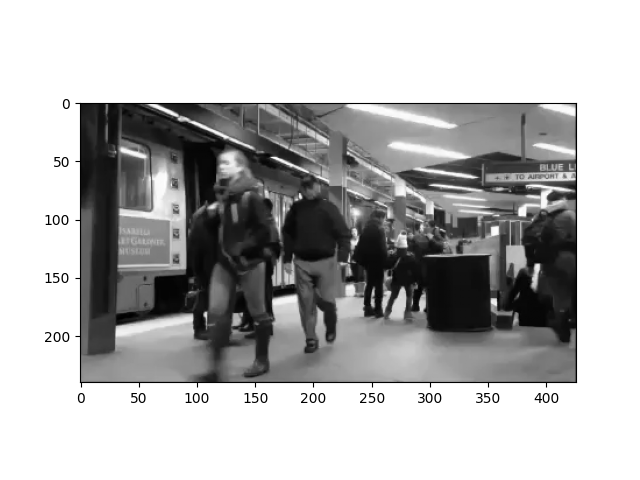# Generating a stimulus from a video¶

This example shows how to use videos as input stimuli for a retinal implant.

A video can be loaded as follows:

stim = p2p.stimuli.videos.VideoStimulus("path-to-video.mp4")


There is an example video that is pre-installed with pulse2percept. You can load it like this.

import pulse2percept as p2p
import numpy as np

video = p2p.stimuli.BostonTrain(as_gray=True)
print(video)

BostonTrain(data=<(102240, 94) np.ndarray>, dt=0.001,
electrodes=[     0      1      2 ... 102237 102238 102239],
shape=(102240, 94), time=<(94,) np.ndarray>,
vid_shape=(240, 426, 94))


There is a lot of useful information in this output.

Firstly, note that vid_shape gives the dimension of the original video in (height, width, the number of frames).

On the other hand, shape gives the dimension of the stimulation which is (the number of electrodes, the number of time steps). This is calculated from flattening the video to (height x width, the number of frames).

The video data is stored as a 2D NumPy array in video.data. You can reshape it to the original video dimensions as follows:

data = video.data.reshape(video.vid_shape)


Then it’s possibly to access individual pixels or frames by indexing into the NumPy array. For example, to plot the first frame of the movie, use:

import matplotlib.pyplot as plt
plt.imshow(data[..., 0], cmap='gray')<matplotlib.image.AxesImage object at 0x7f17fa56e290>


## Preprocessing a video¶

A VideoStimulus object comes with a number of methods to process a video before it is passed to an implant. Some examples include:

For a complete list, check out the documentation for VideoStimulus.

Let’s do some processing for our example video. Firstly, let’s play the video so that we know what it looks like originally. (It might take a couple of seconds for the video to appear, because it needs to be converted to HTML & JavaScript code first.)

video.play()